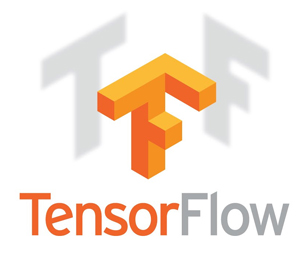Deep Learning with Tensorflow & Keras

Venue : RND Consultancy Services, Serampore, Chatra, Hooghly, WB. Phone : +919830546476 / +918240819346

Deep Learning Course will give you all the knowledge needed to work on Deep Learning libraries like Keras and Tensorflow. In this training we will learn about what is AI, ML, explore neural networks, understand deep learning frameworks, and implement various machine learning algorithms using Deep Networks. We will also explore how different layers in neural networks do data abstraction and feature extraction using Deep Learning.

Anybody interested in Deep Learning can take this Training. Though knowledge of following will be a plus point: Python programming ,Machine Learning,Neural Networks

Introduction to Deep Learning

Why Deep Learning?

What is a neural network?

Reasons to go Deep

Choice of Deep Net

Neural Network

Introduction to Artificial Neural Networks

Feedforward Neural networks

Backpropagation

Activation functions

ML

Overview of Python Programming

Introduction to python

Python data types

Functions

Classes

Module

Using Numpy and Pandas

Introduction to Numpy

Creating N-Dimensional numpy arrays

Array mathematics

Slicing N-Dimensional array

Introduction to pandas

Working with pandasSeries

Working with pandas Dataframe

Deep Learning Models

Restricted Boltzmann Machines

Deep Belief Nets

Convolutional Networks

Recurrent Nets

Additional Deep Learning Models

Autoencoders

Recursive Neural Tensor Nets

Deep Learning Software Libraries I (TensorFlow)

Introduction to TensorFlow

HelloWorld with TensorFlow

Basic computation with TensorFlow

Deep Learning Software Libraries II (Keras)

Introduction to Keras

Keras vs TensorFlow

Building Basic models with Keras

Convolutional Neural Networks (CNN)

CNN History

Understanding CNNs

CNN Application using Keras

Recurrent Neural Networks (RNN)

Intro to RNN Model

Long Short-Term memory (LSTM)

Recursive Neural Tensor Network Theory

Unsupervised Learning

Applications of Unsupervised learning

Restricted Boltzmann Machine

FIND US

Serampore, Chatra, Hooghly, West Bengal, India.
Phone: +919830546476 / +918240819346

E-mail: rndconsultancyservice@gmail.com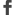Facebook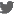Twitter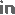LinkedInGoogle+Flicker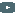YoutubePinterest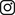Instagram